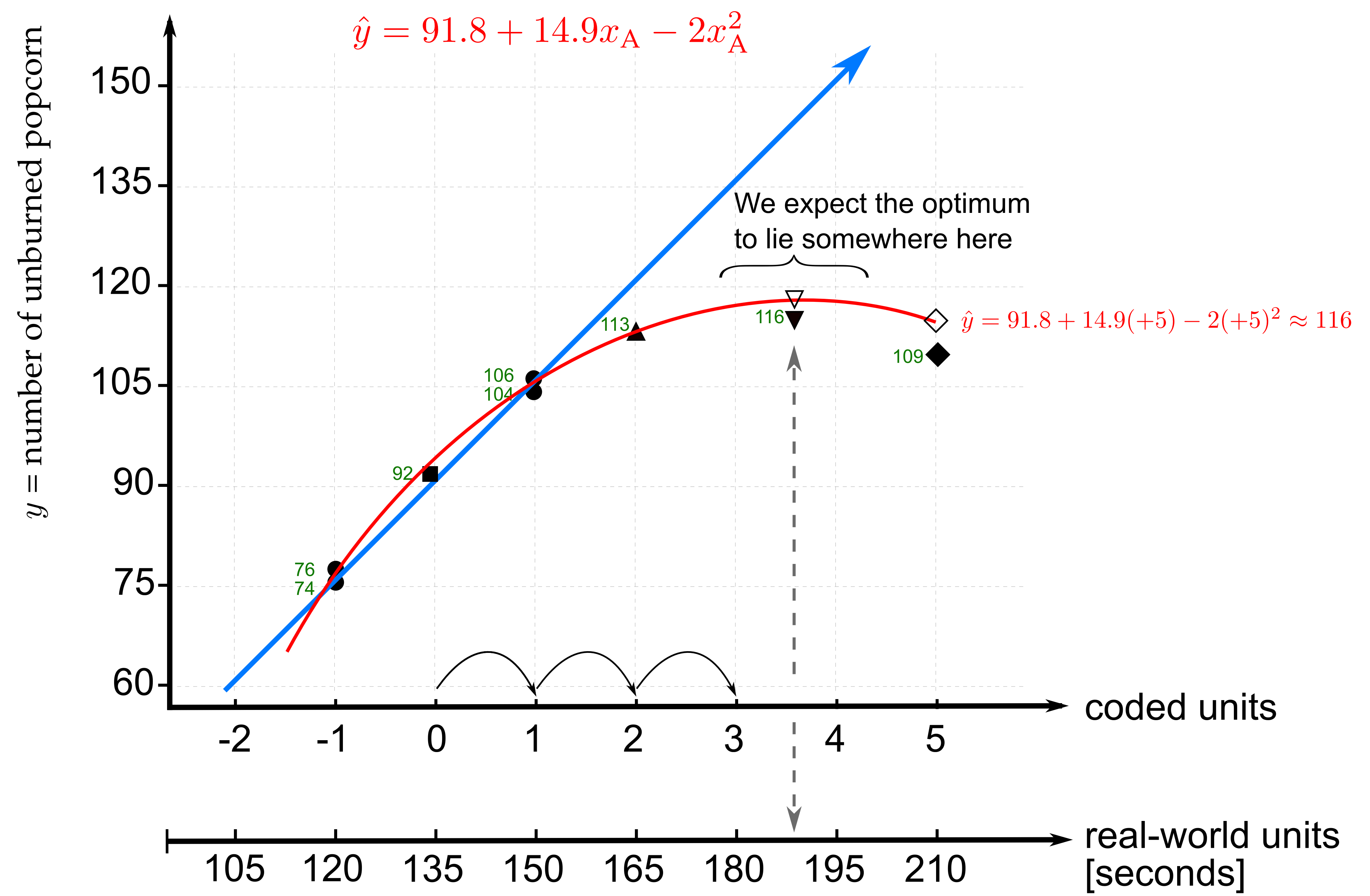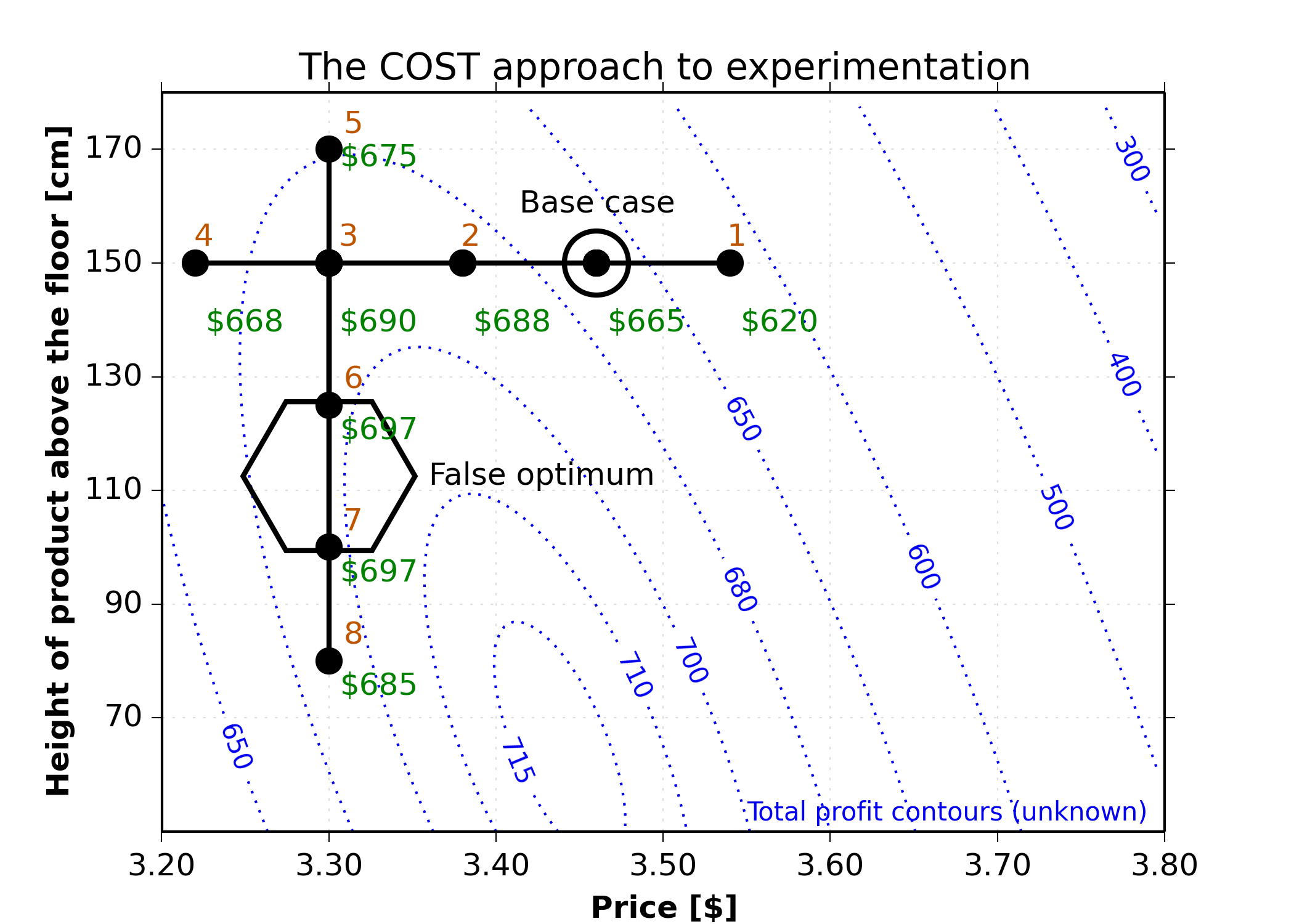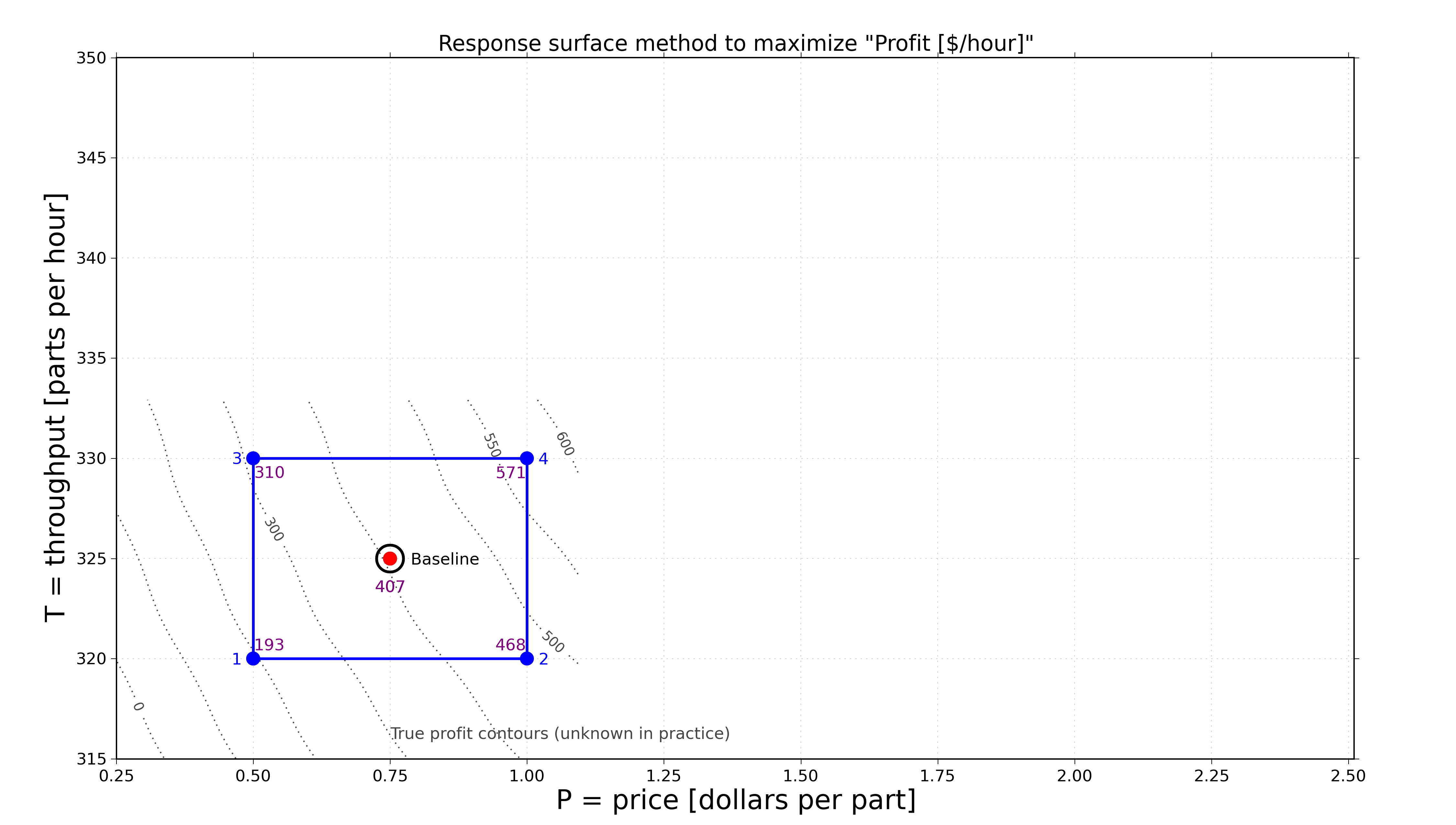## Supplment for Coursera MOOC: Experimentation for Improvement

#### Create your own popcorn (experiments)

Factor A: cooking time seconds    Outcome $$y$$ = amount of unburned popcorn made: ____#### Making the most profit selling a product in a grocery store

 Factor A: price $Factor B: height above the ground cm Outcome $$y$$ = amount of profit made:$ ___ per hour#### Response surface optimization for manufacturing a product

 Factor P: price $per part Factor T: throughput parts per hour Outcome $$y$$ = amount of profit made:$ ____ per hour#### Response surface optimization for coagulation of waste water

 Factor D: dosage (mg/L) typical values lie between 0 and 40 Factor P: pH (dimensionless) typical values lie between 4 and 8.5 Factor B: basicity (scaled) values lie between 0 and 1
Outcome $$y$$ = residue concentration: ____ mg/L (with the aim to minimize it; a value of NaN indicates invalid operation)